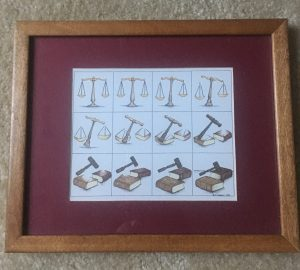Learn the 4 Things You Must Know Before Taking the GMAT!   Download it Now!

Sometimes we overcomplicate GMAT algebra questions.

Actually, let me rephrase that. Sometimes we see what looks to be a challenging GMAT algebra question, we freak out, we convince ourselves that we don’t even know where to begin, and we all but throw in the towel.

Ever been there?

Don’t let that be your reality any longer. I have a methodology (more of a mindset, really) that I think will help you.

## Getting From Point A to Z on the GMAT

My dad was a successful trial lawyer. He had this picture hanging in his office:This picture illustrates the “Art of Transformation” mindset on GMAT Algebra questions

As a kid, I remember thinking it was really cool. Here was this picture of the scales of justice in the upper left, and after just a few simple modifications to the picture at each step, it “magically” turned into a gavel and books!

Pretty neat, right?

So what does this have to do with the GMAT?

You can take the same approach on certain GMAT algebra questions. In fact, here’s the tip for you:

### GMAT Tip: Whenever you feel stuck on a GMAT algebra question, start with the end in mind.

What does that mean?

Imagine you were the artist of the picture above. You know you want the scales of justice to turn into a gavel and books. So how do you get there? What elements of the original picture must be retained at each step? What needs to be changed?

The same methodology and mindset applies on the GMAT sometimes. You’re given one set of information, and you need to manipulate that information to solve for something else. That “something else” is the end result. The question is, how do you get from point A to point Z? In other words, how do you turn the information you’re given into the information you’re trying to solve for?

You need to learn to think like the artist of this picture. Start with the end in mind, and the steps will just sort of fall into place.

## Application Example

Consider this example from The Official Guide for GMAT Review 2017 (p.162 #89):

If mn ≠ 0 and 25 percent of n equals 37½ percent of m, what is the value of 12n/m?

(A) 18
(B) 32/3
(C) 8
(D) 3
(E) 9/8

You’re given some initial information and you want it to eventually “look like” 12n/m. That’s the end result. So how do you get there?

As a starting point, it’s helpful to translate the word problem into an algebraic equation. It’s given that 25% of n (written as 0.25n) equals 37.5% of m (written as 0.375m). So mathematically, we’re told that 0.25n = 0.375m.

Think of that as the starting picture in the upper left. And we eventually want it to look like 12n/m. So how do we proceed? What needs to change from the original equation? What needs to be retained?

• Step 1: Notice that the end result doesn’t have any decimals in it. So a good starting point is to multiply both sides by 100 to get rid of the decimals. Now we have 25n = 37.5m.
• Step 2: Decimals are still annoying, and there aren’t any in the end result we’re going after, so let’s further multiply both sides by 2. Now we have 50n = 75m.
• Step 3: Looking ahead, we eventually want there to be a n/m term, right? So let’s isolate n/m on one side of the equation. First, divide both sides by 50 so that n = 75m/50. Then divide both sides by m so that n/m = 75/50. Reducing that, we have n/m = 3/2.
• Step 4: The question isn’t asking us to solve for just n/m, but rather 12n/m. So multiply both sides by 12, resulting in 12n/m = 12(3/2) = 18.

Done! The correct answer is A, 18.

It may not have been immediately obvious how to get from Point A (25%(n) = (37.5%)m) to Point Z (12n/m = 18), but if you step back and just manipulate small parts of the equation at each step keeping the desired end result in mind, everything just falls into place.

Now if only we could represent this problem in graphical form, it would make such a nice picture!